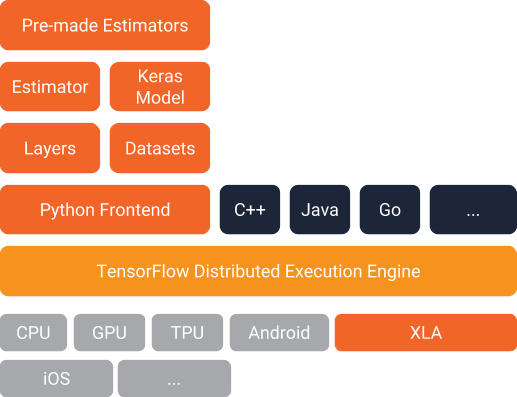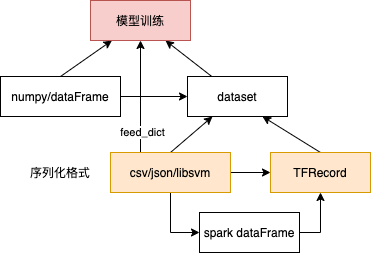2021年08月18日

## 简介

TensorFlow，这是个很形象的比喻，意思是 张量(Tensor)在神经网络中流动(Flow)。

1. 在数学中，张量是一种几何实体(对应的有一个概念叫矢量)，广义上可以表示任何形式的数据。在NumPy等数学计算库或TensorFlow等深度学习库中，我们通常使用多维数组来描述张量，所以不能叫做矩阵，矩阵只是二维的数组，张量所指的维度是没有限制的。线性代数/矩阵的几何意义 未读完
2. 张量这一概念的核心在于，它是一个数据容器。它包含的数据几乎总是数值数据，因此它是数字的容器。
3. 在物理实现时（TensorFlow）是一个句柄，它存储张量的元信息以及指向张量数据的内存缓冲区指针。
4. 张量是执行操作时的输入输出数据，用户通过执行操作来创建或计算张量，张量的形状不一定在编译时确定，可以在运行时通过形状推断计算出。

TensorFlow 使用库模式（不是框架模式），工作形态是由用户编写主程序代码，调用python或其它语言函数库提供的接口实现计算逻辑。用户部署和使用TensorFlow 时，不需要启动专门的守护进程，也不需要调用特殊启动工具，只需要像编写普通本地应用程序那样即可上手。## 核心概念

TensorFlow on Kubernetes的架构与实践

### 数据节点

Tensorflow底层最核心的概念是张量，计算图以及自动微分。

1. Tensor
2. Variable，特殊的张量， 维护特定节点的状态，一般对应w、b，模型的复杂、变大即 tf.Variable 变多，且在分布式场景下由各个节点共享。tf.Variable 方法是Op，返回时是变量。与`session.run(tf.global_variables_initializer())` 配合使用 进行真正的初始化。与Tensor 不同在于
3. 普通Tensor 的生命周期通常随依赖的计算完成而结束，内存也随即释放。
4. 变量常驻内存， 在每一步训练时不断更新其值，以实现模型参数的更新。
5. placeholder 占位符，一般对应 x、 y_hat、需要变化的超参数等，使用 `session.run(...,feed_dict={xx:xx})` 为占位符赋值

### 计算图

``````x = tf.constant("hello")
y = tf.constant("world")
z = tf.strings.join([x,y],separator=" ")
print(z)
# output
# Tensor("StringJoin:0", shape=(), dtype=string)
``````

### 会话

Session 提供求解张量和执行操作的运行环境，它是发送计算任务的客户端，所有计算任务都由它分发到其连接的执行引擎（进程内引擎）完成。

``````# 创建会话 target =会话连接的执行引擎（默认是进程内那个），graph= 会话加载的数据流图，config= 会话启动时的配置项
sess = tf.session(target=...,graph=...,config=...)
# 估算张量或执行操作。 Tensor.eval 和 Operation.run 底层都是 sess.run
sess.run(...)
# 关闭会话
sess.close()
``````

``````import tensorflow as tf
a = tf.constant(5.0)
b = tf.constant(6.0)
c = a * b
sess = tf.Session()
print(sess.run(c))
``````

## demo

``````# model parameters
W = tf.Variable(0.3,  tf.float32)
b = tf.Variable(-0.3, tf.float32)
# model inputs & outputs
x = tf.placeholder(tf.float32)
y = tf.placeholder(tf.float32)
# the model
out = W * x + b
# loss function
loss = tf.reduce_sum(tf.square(out - y))
# optimizer
train = optimizer.minimize(loss)
# training data
x_train = np.random.random_sample((100,)).astype(np.float32)
y_train = np.random.random_sample((100,)).astype(np.float32)
# training
init = tf.global_variables_initializer()
with tf.Session() as sess:
sess.run(init)
for i in range(1000):
sess.run(train, {x:x_train, y:y_train})
current_loss = sess.run(loss, {x:x_train, y:y_train})
print("iter step %d training loss %f" % (i, current_loss))
print(sess.run(W))
print(sess.run(b))
``````

``````df = pd.read_csv('data.csv',names=['square', 'bedrooms', 'price'])
ones = pd.DataFrame({'ones': np.ones(len(df))})# ones是n行1列的数据框，表示x0恒为1
df = pd.concat([ones, df], axis=1)  # 根据列合并数据
``````

| |ones| square| bedrooms| price| |—|—|—|—|—| |0| 1.0| 0.130010| -0.223675| 0.475747| |1| 1.0| -0.504190| -0.223675| -0.084074|

``````X_data = np.array(df[df.columns[0:3]])
y_data = np.array(df[df.columns[-1]]).reshape(len(df), 1)
# 输入 X，形状[47, 3]
X = tf.placeholder(tf.float32, X_data.shape)
# 输出 y，形状[47, 1]
y = tf.placeholder(tf.float32, y_data.shape)
# 权重变量 W，形状[3,1]
W = tf.get_variable("weights", (X_data.shape, 1), initializer=tf.constant_initializer())
# 假设函数 h(x) = w0*x0+w1*x1+w2*x2, 其中x0恒为1
# 推理值 y_pred  形状[47,1]
y_pred = tf.matmul(X, W)
# 损失函数采用最小二乘法，y_pred - y 是形如[47, 1]的向量。
loss_op = 1 / (2 * len(X_data)) * tf.matmul((y_pred - y), (y_pred - y), transpose_a=True)
train_op = opt.minimize(loss_op)
with tf.Session() as sess:
# 初始化全局变量
sess.run(tf.global_variables_initializer())
# 开始训练模型，
# 因为训练集较小，所以每轮都使用全量数据训练
for e in range(1, epoch + 1):
sess.run(train_op, feed_dict={X: X_data, y: y_data})
``````

## 数据读取

1. 数据源： csv/TFRecord/Tensor/numpy/dataFrame/自定义格式
2. 读取方式：批量，多线程
3. 读取的数据如何给到模型使用： 作为 tensor 给feed_dict；新的Dataset `y_hat = model(x)`### feed_dict 读取csv（不建议）

``````file_queue = tf.train.string_input_producer(["xx.csv"])
record_defaults=[["1.0"], ["1"],["2.0"]]
column1, column2, column3 = tf.decode_csv(content, record_defaults)
# column1_batch, column2_batch, column3_batch = tf.train.batch([column1, column2], batch_size=9, num_threads=1, capacity=9)
features = tf.stack([column1, column2])
...
with tf.Session() as sess:
sess.run(...,feed_dict = {X = features, Y = column3})
``````

### Dataset

feed-dict 被吐槽太慢了 ，如何在TensorFlow上高效地使用Dataset推荐使用dataset API的原因在于，他提供了一套构建数据Pipeline的操作，包括以下三部分

1. 从数据源构造dataset（支持TFRecordDataset，TextLineDataset, CsvDataset等方式)
2. 数据处理操作 (通过map操作进行转化，tfrecord格式需要对Example进行解析)
3. 迭代数据 （包括batch, shuffle, repeat, cache等操作）

Tensorflow封装了一组API来处理数据的读入，它们都属于模块 tf.data

1. 从numpy 读取
``````  # create a random vector of shape (100,2)
x = np.random.sample((100,2))
# make a dataset from a numpy array
dataset = tf.data.Dataset.from_tensor_slices(x)
``````
2. 从磁盘/内存中获取源数据，比如直接读取特定目录的下的 xx.png 文件，使用 dataset.map 将输入数据转为 dataset 要求
3. 读入TFrecords数据集，`dataset = tf.data.Dataset.TFRecordDataset("dataset.tfrecords")`

Iterator 是对应的Dataset迭代器。如果 Dataset 是一个水池的话，那么它其中的数据就好比是水池中的水，Iterator 可以把它当成是一根水管。

``````features, labels = (np.random.sample((100,2)), np.random.sample((100,1)))
dataset = tf.data.Dataset.from_tensor_slices((features,labels))
# 描述 了数据的来源 batch size 等
dataset = tf.data.Dataset.from_tensor_slices((features,labels)).repeat().batch(BATCH_SIZE)
# 创建迭代器
iter = dataset.make_one_shot_iterator()
x, y = iter.get_next()
# 定义模型
net = tf.layers.dense(x, 8, activation=tf.tanh) # pass the first value
net = tf.layers.dense(net, 8, activation=tf.tanh)
prediction = tf.layers.dense(net, 1, activation=tf.tanh)
loss = tf.losses.mean_squared_error(prediction, y)
with tf.Session() as sess:
sess.run(tf.global_variables_initializer())
for i in range(EPOCHS):
_, loss_value = sess.run([train_op, loss])
``````

dataset 由 elements 组成，elements 由component 组成

1. elements include tuple, dict, NamedTuple, and OrderedDict。dataset 实现了python Iterable，一次 `next()` 输出一个element。比如 `[<component,component>,...] [dict(component,component),]`
2. Individual components of the structure can be of any type representable by tf.TypeSpec, including tf.Tensor, tf.sparse.SparseTensor, tf.RaggedTensor, tf.TensorArray, or tf.data.Dataset.

### TFRecord 文件

TFRecord是Tensorflow训练和推断标准的数据存储格式之一，将数据存储为二进制文件（二进制存储具有占用空间少，拷贝和读取（from disk）更加高效的特点），而且不需要单独的标签文件了，其本质是一行行字节字符串构成的样本数据。一条TFRecord数据代表一个Example，一个Example就是一个样本数据，每个Example内部由一个字典构成`<key,Feature>`，key为字段名，Feature为字段名所对应的数据，Feature有三种数据类型：ByteList、FloatList，Int64List。TFRecord并不是一个self-describing的格式，也就是说，tfrecord的write和read都需要额外指明schema。

1. 小规模生成TFRecord： python 代码将图像、文本等写入tfrecords文件需要建立一个writer对象，创建这个对象的是函数 `tf.python_io.TFRecordWriter`
2. 大规模生成TFRecord：如何大规模地把HDFS中的数据直接喂到Tensorflow中呢？Tensorflow提供了一种解决方法：spark-tensorflow-connector，支持将spark DataFrame格式数据直接保存为TFRecords格式数据。

TensorFlow 指南：读取自定义文件和记录格式

## 为计算图加入控制逻辑

TensorFlow 中的 Control Flow

TensorFlow 记录每个运算符的依赖，根据依赖进行调度计算。数据流图上节点的执行顺序的实现参考了拓扑排序的设计思想

1. 以节点名称作为关键字，入度作为值，创建一张散列表，并将此数据流图上的所有节点放入散列表中。
2. 为此数据流图创建一个可执行节点队列，将散列表中入度为0的节点加入到该队列，并从散列表中删除这些节点
3. 依次执行该队列中的每一个节点，执行成功后将此节点输出指向的节点的入度值减1，更新散列表中对应节点的入度值
4. 重复2和3，知道可执行节点队列变为空。 这也导致了 运算符执行顺序可能和 代码顺序不同
``````var = tf.Variable(...)
top = var * 2
out = top + bot
``````

``````var = tf.Variable(...)
top = var * 2
with tf.control_dependencies([top]):
out = top + bot
``````

## 可视化

1. summary，在定义计算图时，在适当位置加上一些summary 操作。 summary 操作输入输出也是张量，只是输出是汇总数据。
2. merge，在训练时可能加入了 多个summary 操作，此时需要使用 tf.summary.merge_all 将这些summary 操作 合成一个操纵
3. run，执行session.run 时，需要通过 tf.summary.FileWrite() 指定一个目录告诉程序把产生的文件放到指定位置，然后使用`add_summary()` 将某一步summary 数据记录到文件中
4. `tensorboard --logdir=path/to/log-directory` 启动tensorboard 然后在浏览器打开页面

1. 可视化数据流图
``````  sess = xx
writer = tf.summary.FileWriter("/tmp/summary/xx",sess.graph)
...
writer.close()
``````
2. 可视化度量指标和模型参数 ```python tf.summary.scalar(‘name’,’tensor’)

for i in range(FLAGs.max_step): summary, acc = sess.run(…) writer.add_summary(summary,i) ```

1. 可视化图形和音频数据
2. 可视化高维数据，用于展示高维embedding 数据的分布情况
3. 可视化性能与资源占用 使用 Profiler 优化 TensorFlow 性能。 除了跟TensorBoard 一起使用外， profiler 也可以单独 执行和分析 tensorflow Profile分析神经网络性能
4. 使用 tf.profiler Function API 的编程模式 ```python tf.profiler.experimental.start('logdir') # 会保存为json 格式文件 # Train the model here tf.profiler.experimental.stop() ```
5. 使用 TensorBoard Keras 回调 (tf.keras.callbacks.TensorBoard) 的编程模式 ```python # Profile from batches 10 to 15 tb_callback = tf.keras.callbacks.TensorBoard(log_dir=log_dir,profile_batch='10, 15') # Train the model and use the TensorBoard Keras callback to collect performance profiling data model.fit(train_data,steps_per_epoch=20,epochs=5,callbacks=[tb_callback]) ```